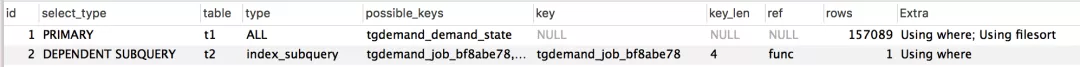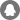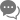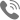### 新睿云

> 知识库 > 记一次 MySQL 的慢查分析

# 记一次 MySQL 的慢查分析

## 定位原因

``SELECT * FROM tgdemand_demand t1WHERE  (    t1.id IN    (      SELECT t2.demand_id      FROM tgdemand_job t2      WHERE (t2.state = 'working' AND t2.wangwang = 'abc')    )    AND    NOT (t1.state = 'needConfirm')  )ORDER BY t1.create_date DESC````SELECT * FROM tgdemand_demand t1 WHERE EXISTS (  SELECT * FROM tgdemand_job t2 WHERE t1.id = t2.demand_id AND (t2.state = 'working' AND t2.wangwang = 'abc')) AND NOT (t1.state = 'needConfirm')ORDER BY t1.create_date DESC;``

## 问题修复

``ids = SELECT t2.demand_idFROM tgdemand_job t2WHERE (t2.state = 'working' AND t2.wangwang = 'abc');SELECT * FROM tgdemand_demand t1WHERE  (    t1.id IN ids    AND    NOT (t1.state = 'needConfirm')  )ORDER BY t1.create_date DESC;``

``demand_ids = Job.objects.filter(wangwang=user['wangwang'], state='working').values_list("demand_id", flat=True)demands = Demand.objects.filter(id__in=demand_ids).exclude(state__in=['needConfirm']).order_by('-create_date')``

``demand_ids = list(Job.objects.filter(wangwang=user['wangwang'], state='working').values_list("demand_id", flat=True))demands = Demand.objects.filter(id__in=demand_ids).exclude(state__in=['needConfirm']).order_by('-create_date')``

``select * from tgdemand_demand t1, (select t.demand_id from tgdemand_job t where t.state = 'working' and t.wangwang = 'abc') t2where t1.id=t2.demand_id and not (t1.state = 'needConfirm')order by t1.create_date DESC``

## 参考资料

http://www.cnblogs.com/zhengyun_ustc/p/slowquery3.html
http://dev.mysql.com/doc/refman/5.5/en/explain-output.html
https://docs.djangoproject.com/en/1.9/ref/models/querysets/QQ咨询在线咨询咨询热线 400-0505-565

{{item.description}}—您的烦恼我们已经收到—